# Electronics basics

### What is resistor??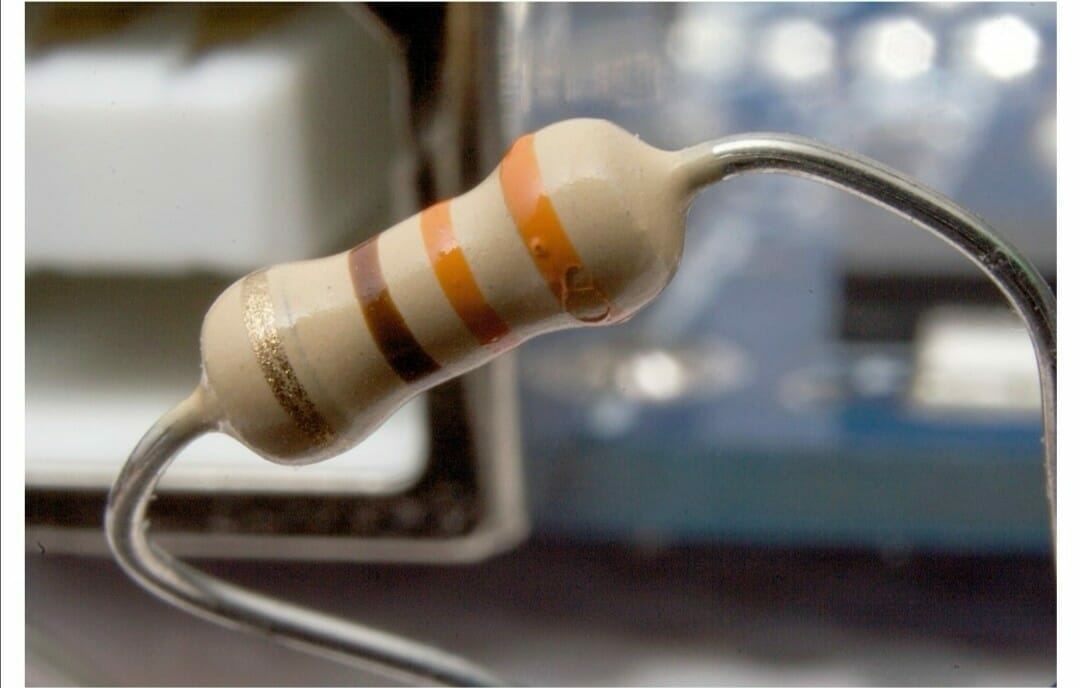A resistor is a passive electronic component that we use in electronic circuits either to limit current through an active component or to reduce voltage in the circuit (by dropping voltage across the resistor).Resistance values is called Ohm.

There are two types of resistors;

• Fixed Resistor
• Variable Resistor

They are also classified according to the material from which they are made.

• Carbon film or metal film resistor
• Wire wound resistor
• Foil resistor
• Grid resistor

### What is transistor?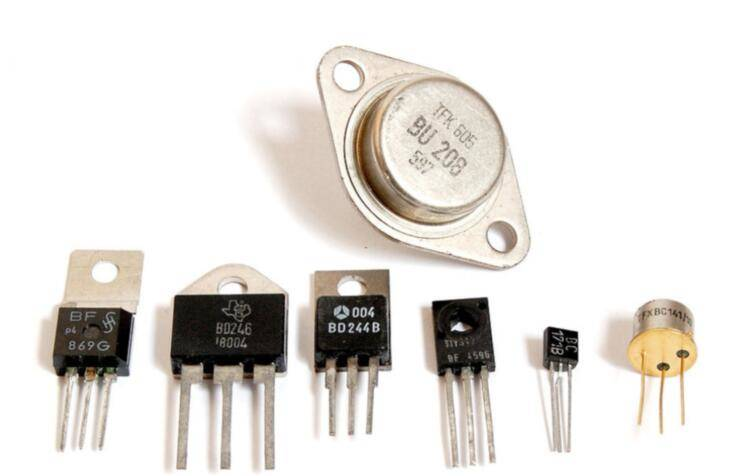A transistor is a semiconductor device used to amplify and switch electronic signals and electrical power. It is composed of semiconductor material with at least three terminals for connection to an external circuit. A voltage or current applied to one pair of the transistors terminals changes the current flowing through another pair of terminals., a transistor can amplify a signal. found embedded in integrated circuits.
Transistor are electrical device, which utilize a sandwich of p and n type of semi conductor materials.
It can be NPN or PNP types
It has got 3 parts :

• emitter
• Base
• Collector.

One of the n type at the left is emitter
Right is collector
And central p type is the base

### What is diode?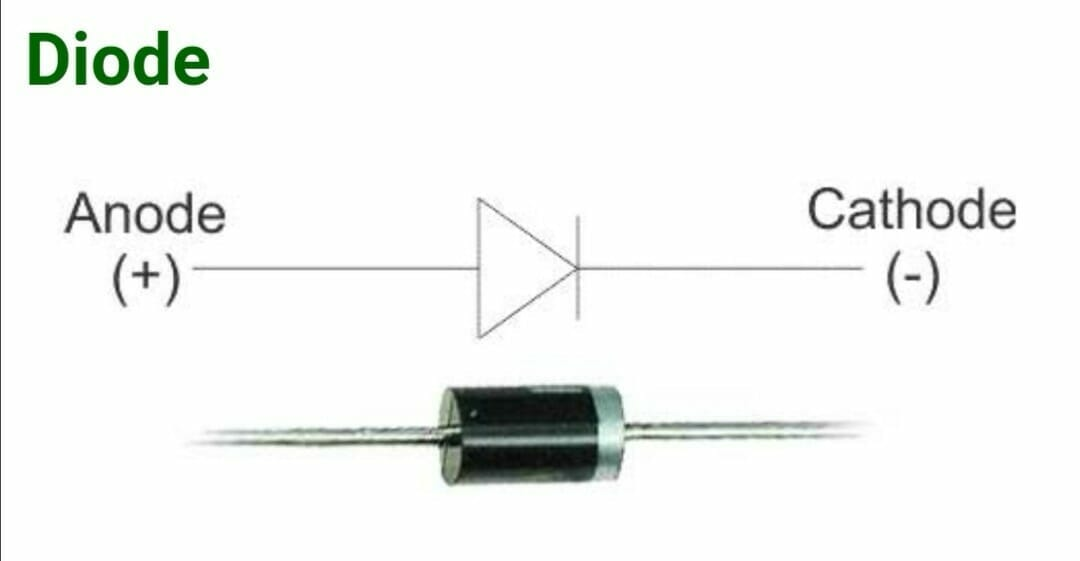A diode is a specialized electronic component with two electrodes called the anode and the cathode. Most diodes are made with semiconductor materials such as silicon, germanium, or selenium. This is about show semiconductor diode working function.
Diodes only allow current to flow in one direction, and they’re always polarized. A diode has two terminals. The positive side is called the anode, and the negative one is called the cathode.
what is it used for?
Diodes can be used as rectifiers, signal limiters, voltage regulators, switches, signal modulators, signal mixers, signal demodulators, and oscillators. The fundamental property of a diode is its tendency to conduct electric current in only one direction.
How do you test a diode?
Set the multimeter to measure ac or dc voltage as required. Turn the dial to Resistance mode
It may share a space on the dial with another function. Connect the test leads to the diode after it has been removed from the circuit.

What is the function of a diode in a circuit?

Main functions. The most common function of a diode is to allow an electric current to pass in one direction (called the diode’s forward direction), while blocking current in the opposite direction (the reverse direction). Thus, the diode can be viewed as an electronic version of a check valve.

### What is a Capacitor?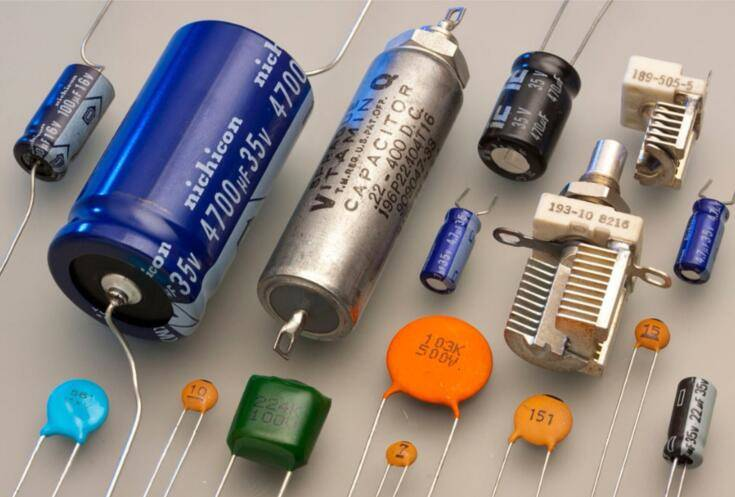A device that stores electrons. The basic capacitor is made up of two conductors separated by an insulator or dielectric.
Depending on how the capacitor is built, the dielectric can be made of paper, plastic, mica, ceramic, glass, a vacuum or any other such non conductive material.
Capacitor come in variety of sizes, shapes, models, or if you so desire they can be manufactured by your specifications.
The value of a capacitor can vary from a fraction of a pico-Farad to more than a micro Farad. Voltage levels can range from a couple to a substantial couple of hundred thousand volts.

What is inductor?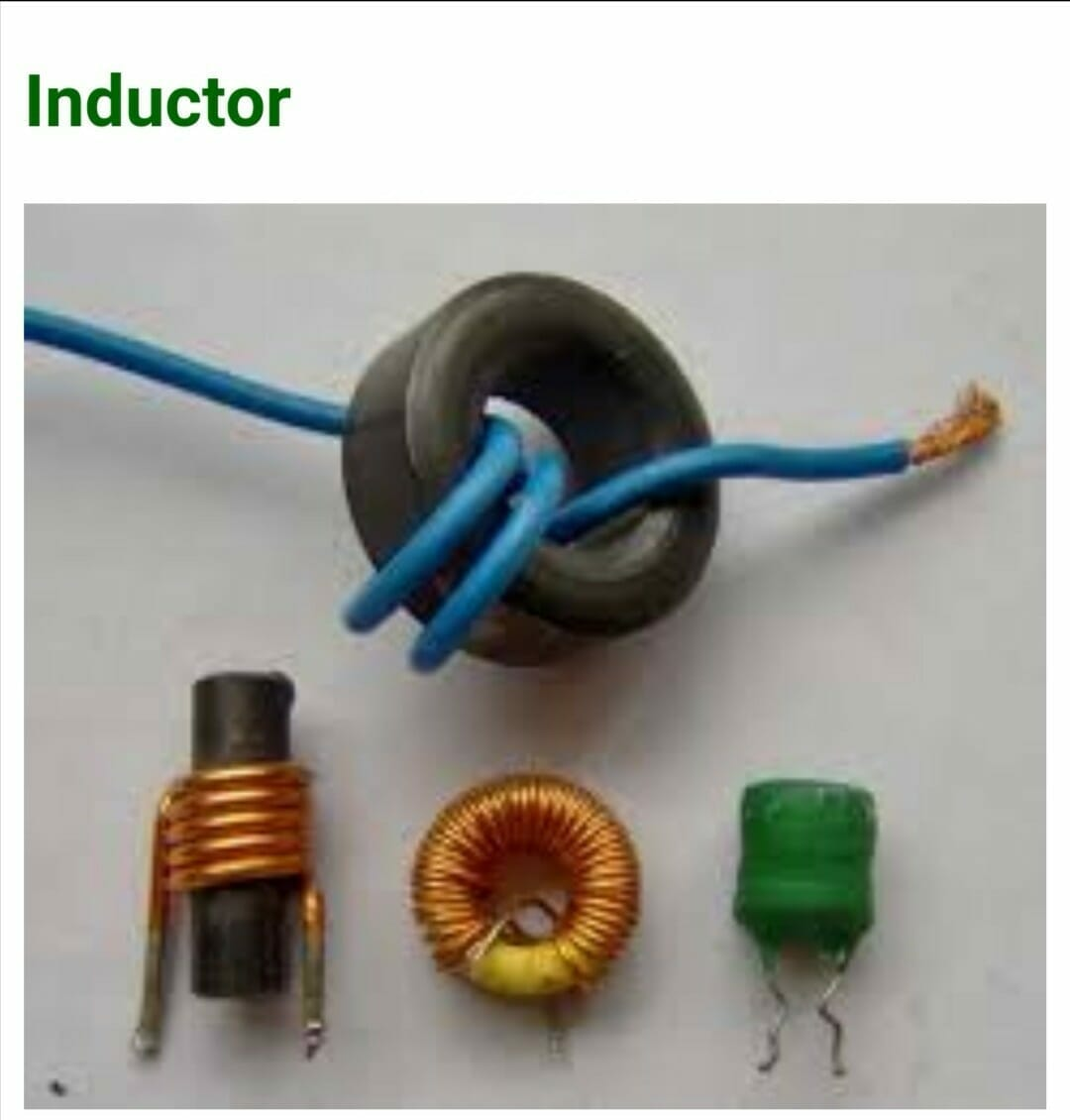Inductors are simply coils of wire.
Inductance is the ability of a coil to establish (or induce) a voltage within itself to oppose changes in current through its windings.
That means when varying current flows through a coil, a voltage is induced within the coil in a direction so as to oppose the change of current through it

Inductance is measured in Henry(s).
A Henry is a measure of the intensity of the magnetic field that is produced.
Typical inductor values used in electronics are in the range of milli Henry (1/1000) and micro Henry (1/1,000,000)
Like capacitors, inductors temporarily store energy.
Unlike capacitors: Inductors store energy in a magnetic field, not an electric field. When the source of electrons is removed, the magnetic field collapses immediately.

The amount of inductance is influenced by a number of factors:

• Number of coil turns.
• Diameter of coil.
• Spacing between turns.
• Size of the wire used.
• Type of material inside the coil.
• Applications of inductors

One of the major applications come in from “mutually coupled” coils where the magnetic field established in one coil, ‘cuts’ through the other coil and hence induces a voltage in the other coil. This is called ‘mutual inductance1. Such coils are widely used in transformers